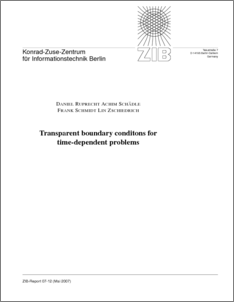Repository: Freie Universität Berlin, Math Department

# Transparent Boundary Conditions for Time-Dependent Problems

Ruprecht, D. and Schaedle, A. and Schmidt, F. and Zschiedrich, L. (2007) Transparent Boundary Conditions for Time-Dependent Problems. Technical Report. Konrad Zuse Zentrum Berlin.Preview

793kB

## Abstract

A new approach to derive transparent boundary conditions (TBCs) for wave, Schrödinger, heat and drift-diffusion equations is presented. It relies on the pole condition and distinguishes between physical reasonable and unreasonable solutions by the location of the singularities of the spatial Laplace transform of the exterior solution. To obtain a numerical algorithm, a Möbius transform is applied to map the Laplace transform onto the unit disc. In the transformed coordinate the solution is expanded into a power series. Finally, equations for the coefficients of the power series are derived. These are coupled to the equation in the interior, and yield transparent boundary conditions. Numerical results are presented in the last section, showing that the error introduced by the new approximate TBCs decays exponentially in the number of coefficients.

Item Type: Monograph (Technical Report) Mathematical and Computer Sciences > Mathematics > Applied Mathematics Department of Mathematics and Computer Science > Institute of Mathematics > Geophysical Fluid Dynamics Group 540 Ulrike Eickers 14 Jul 2009 12:44 03 Mar 2017 14:40

Repository Staff Only: item control page